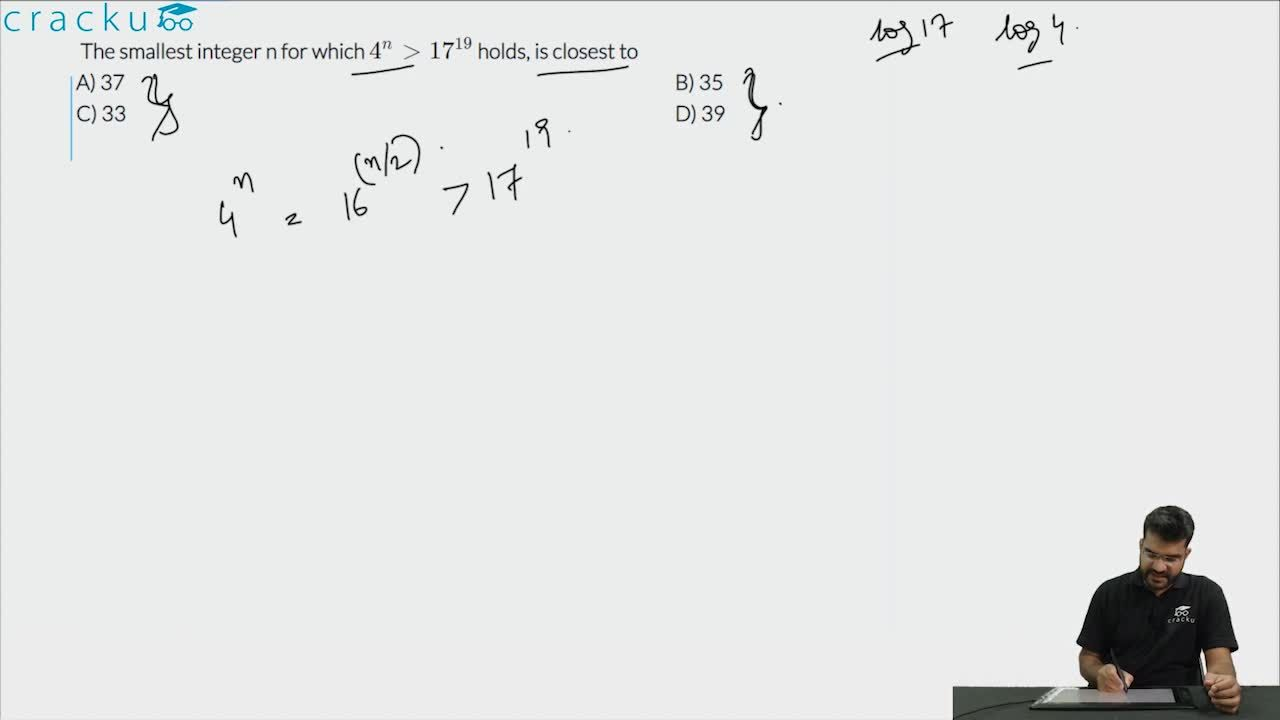Question 90

# The smallest integer n for which $$4^{n} > 17^{19}$$ holds, is closest to

Solution

$$4^{n} > 17^{19}$$

$$\Rightarrow$$ $$16^{n/2} > 17^{19}$$

Therefore, we can say that n/2 > 19

n > 38

Hence, option D is the correct answer.

### View Video Solution